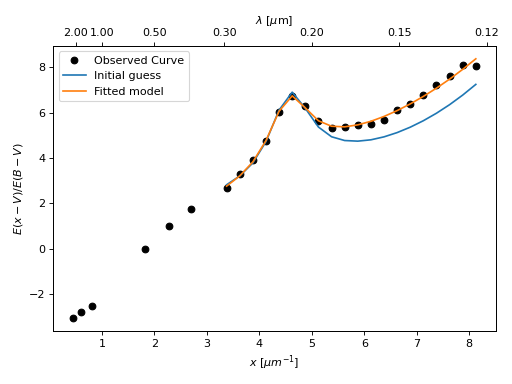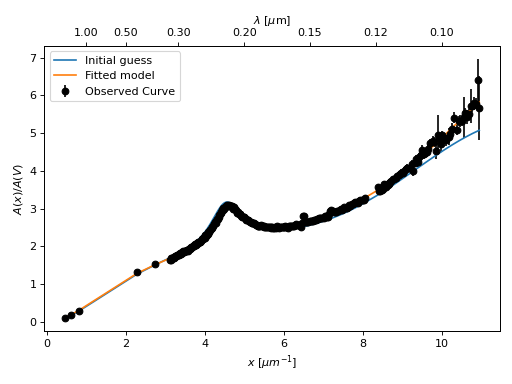# Fit Extinction Curves¶

The dust_extinction package is built on the astropy.modeling package. Fitting is done in the standard way for this package where the model is initialized with a starting point (either the default or user input), the fitter is chosen, and the fit performed.

## Example: FM90 Fit¶

In this example, the FM90 model is used to fit the observed average extinction curve for the LMC outside of the LMC2 supershell region (G03_LMCAvg dust_extinction model).

import warnings
import matplotlib.pyplot as plt
import numpy as np

from astropy.modeling.fitting import LevMarLSQFitter
import astropy.units as u

from dust_extinction.averages import G03_LMCAvg
from dust_extinction.shapes import FM90

# get an observed extinction curve to fit
g03_model = G03_LMCAvg()

x = g03_model.obsdata_x / u.micron
# convert to E(x-V)/E(B0V)
y = (g03_model.obsdata_axav - 1.0) * g03_model.Rv
# only fit the UV portion (FM90 only valid in UV)
(gindxs,) = np.where(x > 3.125 / u.micron)

# initialize the model
fm90_init = FM90()

# pick the fitter
fit = LevMarLSQFitter()

# fit the data to the FM90 model using the fitter
#   use the initialized model as the starting point

# ignore some warnings
#   UserWarning is to avoid the units of x warning
with warnings.catch_warnings():
warnings.simplefilter("ignore", category=UserWarning)
g03_fit = fit(fm90_init, x[gindxs].value, y[gindxs])

# plot the observed data, initial guess, and final fit
fig, ax = plt.subplots()

ax.plot(x, y, "ko", label="Observed Curve")
ax.plot(x[gindxs], fm90_init(x[gindxs]), label="Initial guess")
ax.plot(x[gindxs], g03_fit(x[gindxs]), label="Fitted model")

ax.set_xlabel("$x$ [$\mu m^{-1}$]")
ax.set_ylabel("$E(x-V)/E(B-V)$")

ax.set_title("Example FM90 Fit to G03_LMCAvg curve")

ax.legend(loc="best")
plt.tight_layout()
plt.show()## Example: P92 Fit¶

In this example, the P92 model is used to fit the observed average extinction curve for the MW (GCC09_MWAvg dust_extinction model). The fit is done using the observed uncertainties that are passed as weights. The weights assume the noise is Gaussian and not correlated between data points.

import warnings
import matplotlib.pyplot as plt

from astropy.utils.exceptions import AstropyWarning
import astropy.units as u

from astropy.modeling.fitting import LevMarLSQFitter

from dust_extinction.averages import GCC09_MWAvg
from dust_extinction.shapes import P92

# get an observed extinction curve to fit
g09_model = GCC09_MWAvg()

# get an observed extinction curve to fit
x = g09_model.obsdata_x / u.micron
y = g09_model.obsdata_axav
y_unc = g09_model.obsdata_axav_unc

# initialize the model
p92_init = P92()

# fix a number of the parameters
#   mainly to avoid fitting parameters that are constrained at
#   wavelengths where the observed data for this case does not exist
p92_init.FUV_lambda.fixed = True
p92_init.SIL1_amp.fixed = True
p92_init.SIL1_lambda.fixed = True
p92_init.SIL1_b.fixed = True
p92_init.SIL2_amp.fixed = True
p92_init.SIL2_lambda.fixed = True
p92_init.SIL2_b.fixed = True
p92_init.FIR_amp.fixed = True
p92_init.FIR_lambda.fixed = True
p92_init.FIR_b.fixed = True

# pick the fitter
fit = LevMarLSQFitter()

# fit the data to the P92 model using the fitter
#   use the initialized model as the starting point
#   accuracy set to avoid warning the fit may have failed

# ignore some warnings
#   UserWarning is to avoid the units of x warning
#   AstropyWarning ignored to avoid the "fit may have been unsuccessful" warning
#   fit is fine, but this means the build of the docs fails
with warnings.catch_warnings():
warnings.simplefilter("ignore", category=UserWarning)
warnings.simplefilter("ignore", category=AstropyWarning)
p92_fit = fit(p92_init, x.value, y, weights=1.0 / y_unc)

# plot the observed data, initial guess, and final fit
fig, ax = plt.subplots()

ax.errorbar(x.value, y, yerr=y_unc, fmt='ko', label='Observed Curve')
ax.plot(x.value, p92_init(x), label='Initial guess')
ax.plot(x.value, p92_fit(x), label='Fitted model')

ax.set_xlabel('$x$ [$\mu m^{-1}$]')
ax.set_ylabel('$A(x)/A(V)$')

ax.set_title('Example P92 Fit to GCC09_MWAvg average curve')

ax.legend(loc='best')
plt.tight_layout()
plt.show()